# The order of $\zeta'/\zeta$

Assume the Riemann Hypothesis. Define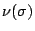for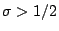to be the greatest lower bound of the numbers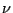for which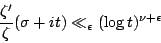holds as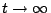for all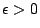. It is a theorem thatis a convex function of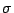which is continuous and decreasing forwith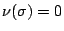for all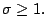It can be shown that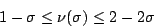for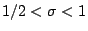. There is an analogous function which can be defined for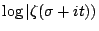and it can be shown that this analogous function is, in fact, equal to. See Titchmarsh for all of these facts. Which bound is corrct?

If the smaller bound is the correct one, then near the half-line we see that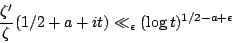for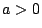. On the other hand,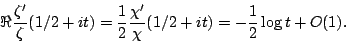Thus, if the smaller bound holds, then there is a jump at 1/2. It could be that the smaller bound holds to the right of the critical-line but that there is radically different behavior on the critical line.

Back to the main index for L-functions and Random Matrix Theory.